The OpenFOAM FoundationdimensionSets Class Reference

## Public Member Functions

dimensionSets (const HashTable< dimensionedScalar > &, const wordList &unitNames)
Construct from all units and set of units to use for inversion. More...

const PtrList< dimensionedScalar > & units () const
Return the units. More...

bool valid () const
Is there a valid inverse of the selected unit. More...

void coefficients (scalarField &) const
(if valid) obtain set of coefficients of unitNames More...

## Detailed Description

Definition at line 76 of file dimensionSets.H.

## ◆ dimensionSets()

 dimensionSets ( const HashTable< dimensionedScalar > & units, const wordList & unitNames )

Construct from all units and set of units to use for inversion.

(writing)

Definition at line 210 of file dimensionSets.C.

Referenced by Foam::writeUnitSet().

Here is the call graph for this function: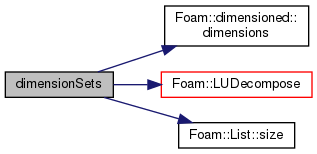Here is the caller graph for this function: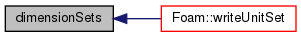## ◆ units()

 const PtrList& units ( ) const
inline

Return the units.

Definition at line 107 of file dimensionSets.H.

Referenced by dimensionSet::write().

Here is the caller graph for this function: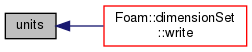## ◆ valid()

 bool valid ( ) const
inline

Is there a valid inverse of the selected unit.

Definition at line 113 of file dimensionSets.H.

Referenced by dimensionSet::write().

Here is the call graph for this function: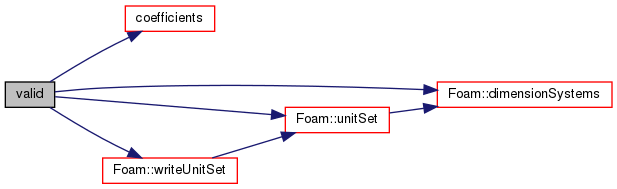Here is the caller graph for this function: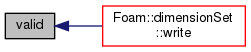## ◆ coefficients()

 void coefficients ( scalarField & exponents ) const

(if valid) obtain set of coefficients of unitNames

Definition at line 253 of file dimensionSets.C.

References Foam::LUBacksubstitute().

Referenced by dimensionSets::valid(), and dimensionSet::write().

Here is the call graph for this function: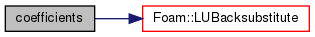Here is the caller graph for this function: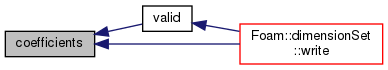The documentation for this class was generated from the following files: